二分类_二分类任务 - CSDN
• 本文为原创文章：http://blog.csdn.net/programmer_wei/article/details/52072939Logistic Regression（逻辑回归）是机器学习中一个非常非常常见的模型，在实际生产环境中也常常被使用，是一种经典的分类模型（不是...
一、逻辑回归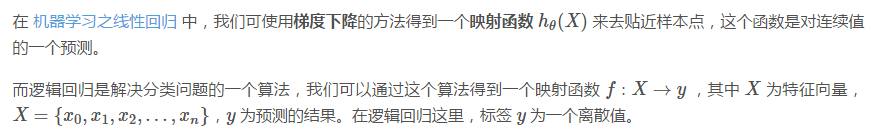二、判定边界当将训练集的样本以其各个特征为坐标轴在图中进行绘制时，通常可以找到某一个 判定边界 去将样本点进行分类。例如：线性判定边界：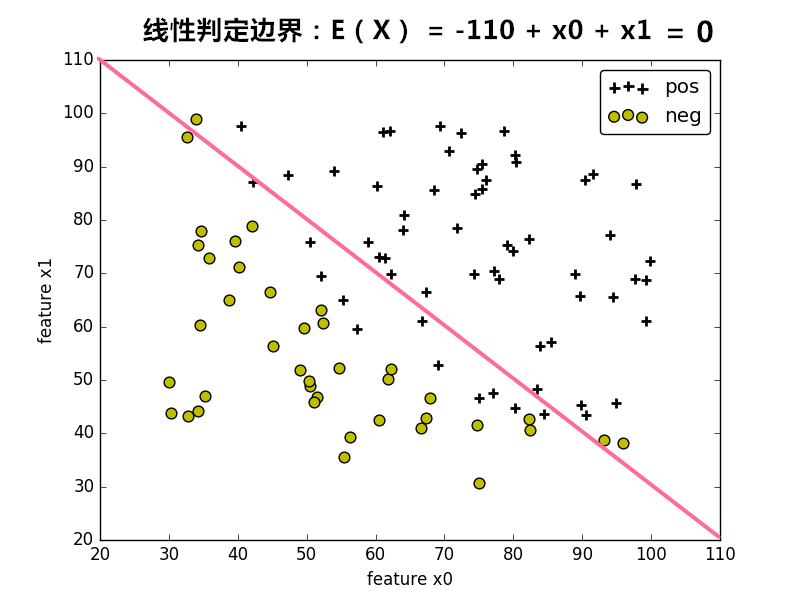非线性判定边界：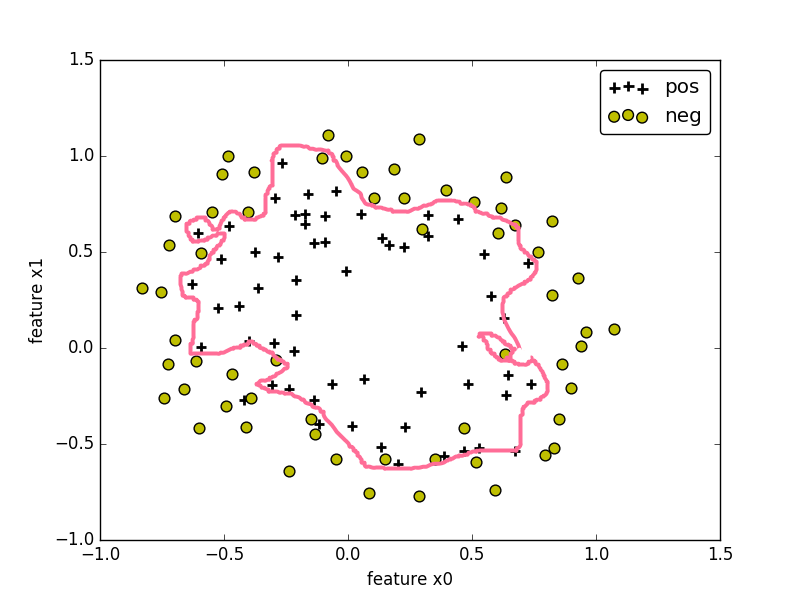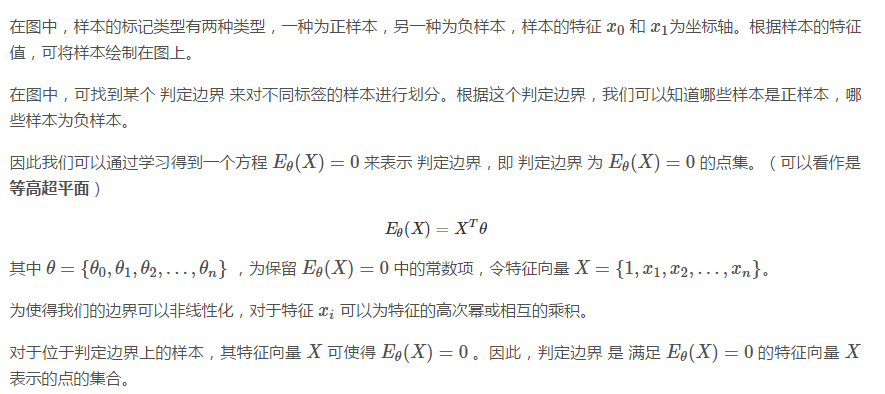三、二分类和sigmoid函数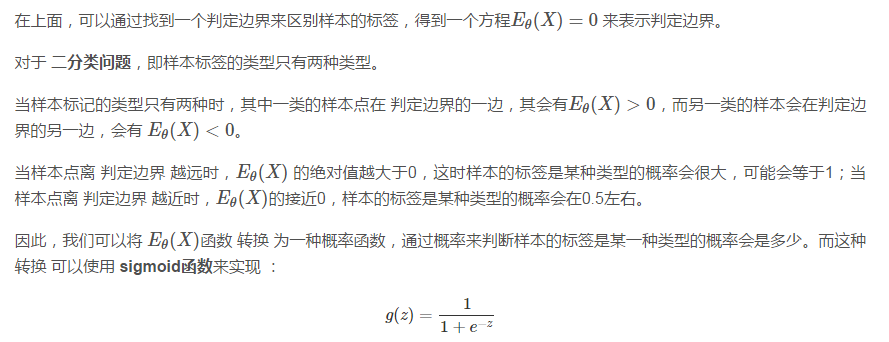sigmoid函数图像如下：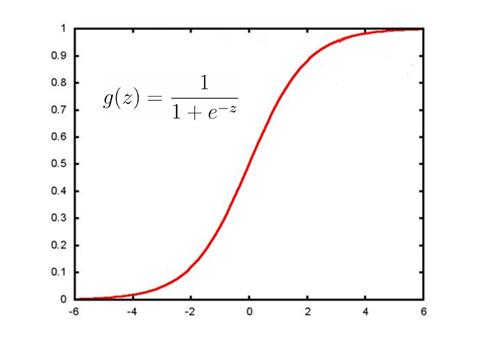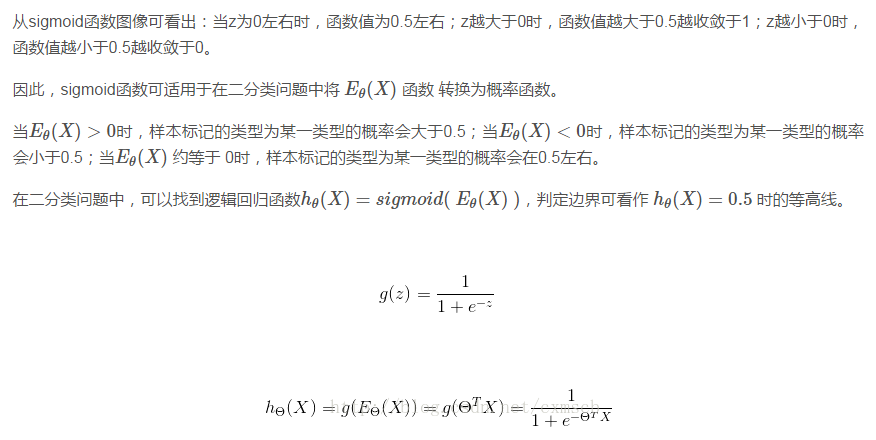四、损失函数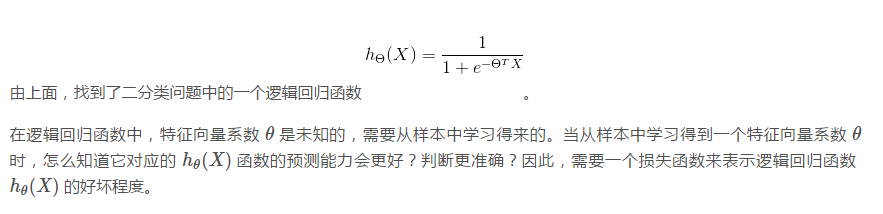1. 定义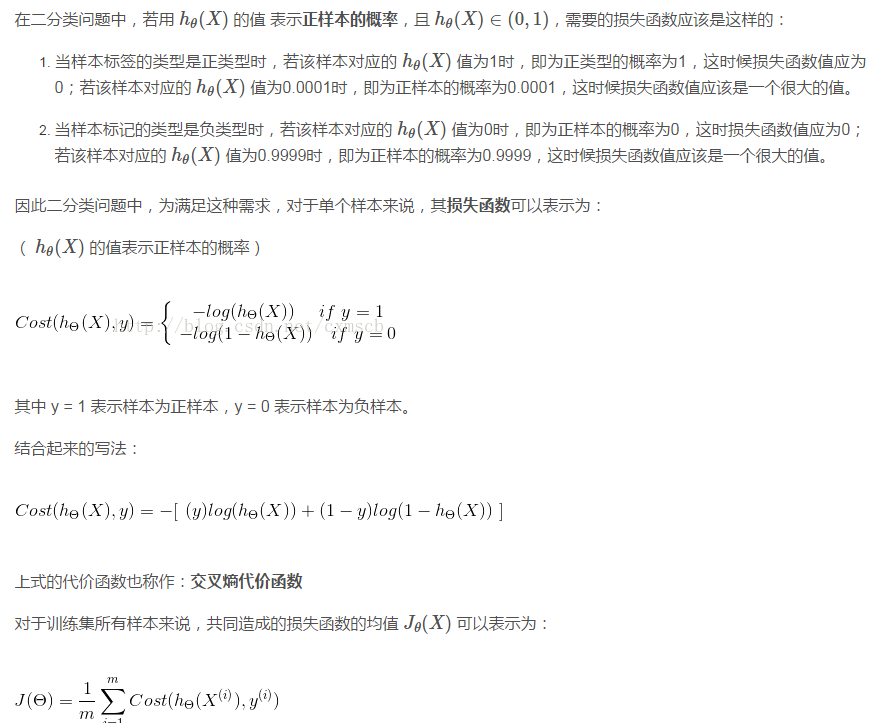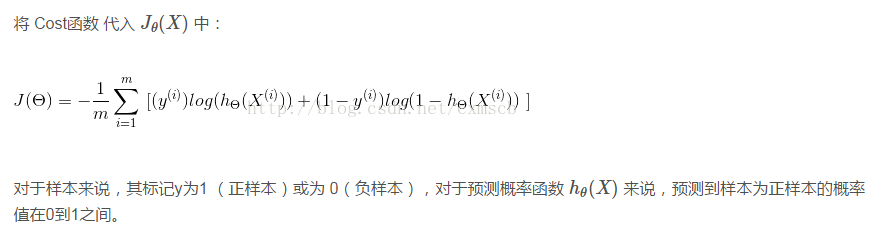2. 极大似然估计上面是一种求损失函数的方式，我们也可以换一种方式来求损失函数，即极大似然估计。用极大似然估计来作为损失函数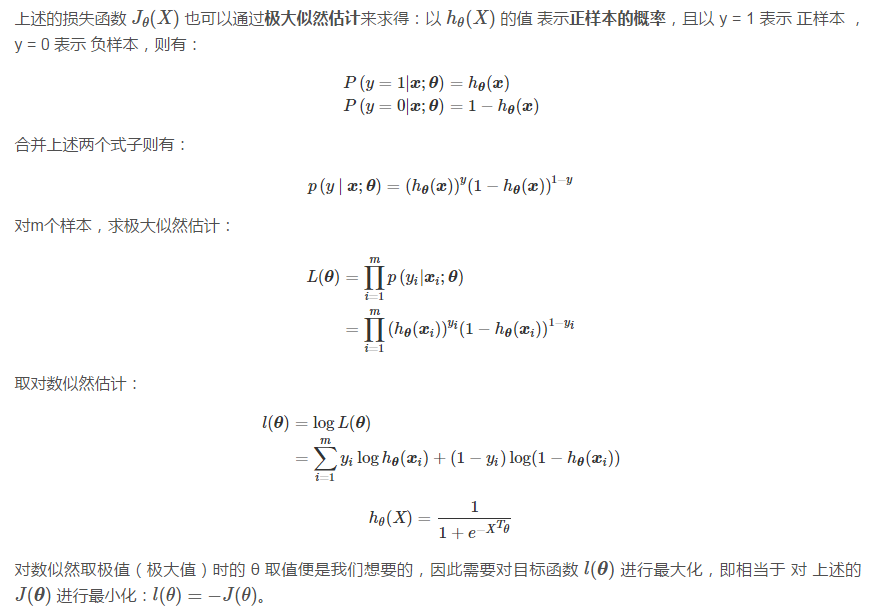3. 正则化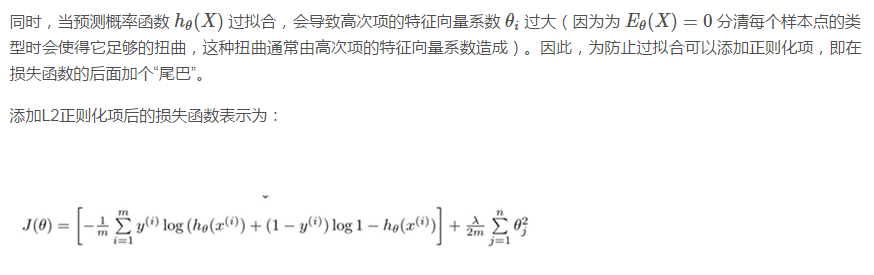五、最小化损失函数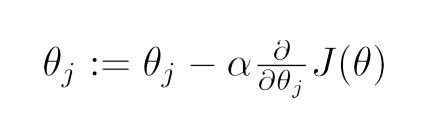同样，上式中的a为学习率（下山步长）。将上式的偏导展开，可得：非正则化的损失函数的偏导：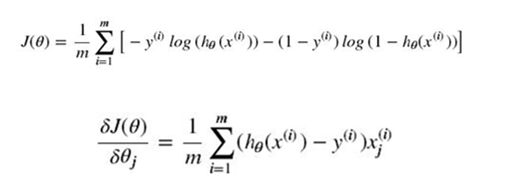含正则化项的损失函数的偏导：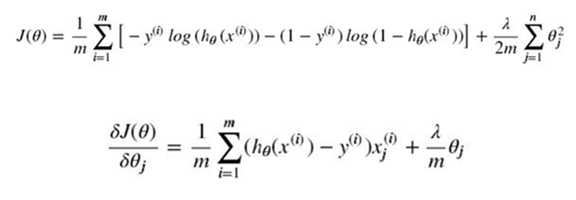其中 λ 为正则化的强度。同线性回归般，可以通过学习率a对特征系数向量中的元素不断进行迭代，直到元素值收敛到某一值即可，这时可以得到损失函数较小时的特征向量系数Θ。六、从二分类过渡到多分类在上面，我们主要使用逻辑回归解决二分类的问题，那对于多分类的问题，也可以用逻辑回归来解决？1. one vs rest由于概率函数 hΘ(X) 所表示的是样本标记为某一类型的概率，但可以将一对一（二分类）扩展为一对多（one vs rest）：将类型class1看作正样本，其他类型全部看作负样本，然后我们就可以得到样本标记类型为该类型的概率p1；然后再将另外类型class2看作正样本，其他类型全部看作负样本，同理得到p2；以此循环，我们可以得到该待预测样本的标记类型分别为类型class i时的概率pi，最后我们取pi中最大的那个概率对应的样本标记类型作为我们的待预测样本类型。2. softmax函数使用softmax函数构造模型解决多分类问题。softmax回归分类器需要学习的函数为 ： （这里下面的公式有问题，括号中的每一项应该都是以e为底的）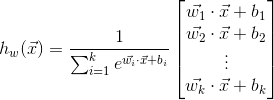其中 k 个 类别的个数 ，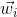和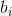为 第 i 个 类别对应的 权重向量 和 偏移标量。其中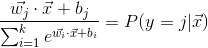可看作样本 X 的标签 为 第 j 个 类别的概率，且有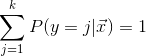。与 logistic回归 不同的是，softmax回归分类模型会有多个的输出，且输出个数 与 类别个数 相等，输出为样本 X 为各个类别的概率 ，最后对样本进行预测的类型为 概率最高 的那个类别。我们需要通过学习得到和，因此建立目标损失函数为：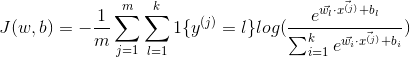上式的代价函数也称作：对数似然代价函数。在二分类的情况下，对数似然代价函数 可以转化为 交叉熵代价函数。其中 m 为训练集样本的个数，k 为 类别的个数，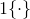为示性函数，当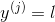为真时，函数值为 1 ，否则为 0 ，即 样本类别正确时，函数值才为 1 。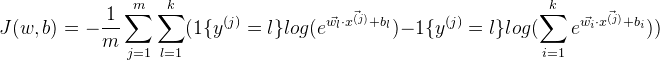继续展开：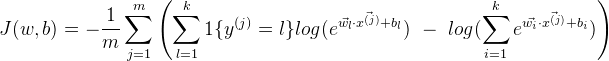通过 梯度下降法 最小化损失函数 和 链式偏导，使用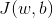对求偏导：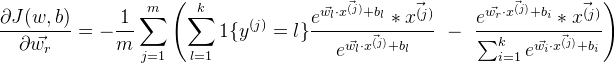化简可得：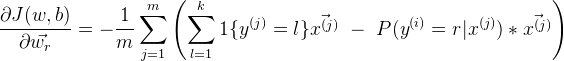再次化简可有：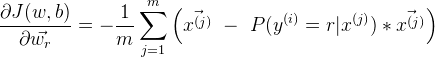因此由 梯度下降法 进行迭代：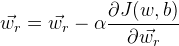同理 通过梯度下降法最小化损失函数也可以得到的最优值。同逻辑回归一样，可以给损失函数加上正则化项。3. 选择的方案当标签类别之间是互斥时，适合选择softmax回归分类器 ;当标签类别之间不完全互斥时，适合选择建立多个独立的logistic回归分类器。4. tensorflow代码示例：使用softmax回归对sklearn中的digit手写数据进行分类import tensorflow as tffrom sklearn.datasets import load_digits
import numpy as npdigits = load_digits()
X_data = digits.data.astype(np.float32)
Y_data = digits.target.reshape(-1,1).astype(np.float32)
print X_data.shape
print Y_data.shape(1797, 64)
(1797, 1)from sklearn.preprocessing import MinMaxScalerscaler = MinMaxScaler()X_data = scaler.fit_transform(X_data)from sklearn.preprocessing import OneHotEncoderY = OneHotEncoder().fit_transform(Y_data).todense() #one-hot编码Ymatrix([[ 1.,  0.,  0., ...,  0.,  0.,  0.],
[ 0.,  1.,  0., ...,  0.,  0.,  0.],
[ 0.,  0.,  1., ...,  0.,  0.,  0.],
...,
[ 0.,  0.,  0., ...,  0.,  1.,  0.],
[ 0.,  0.,  0., ...,  0.,  0.,  1.],
[ 0.,  0.,  0., ...,  0.,  1.,  0.]])print Y.shape(1797, 10)
1797batch_size = 10 # 使用MBGD算法，设定batch_size为10def generatebatch(X,Y,n_examples, batch_size):
for batch_i in range(n_examples // batch_size):
start = batch_i*batch_size
end = start + batch_size
batch_xs = X[start:end, :]
batch_ys = Y[start:end]
yield batch_xs, batch_ys # 生成每一个batchtf.reset_default_graph()
tf_X = tf.placeholder(tf.float32,[None,64])
tf_Y = tf.placeholder(tf.float32,[None,10])tf_W_L1 = tf.Variable(tf.zeros([64,10]))
tf_b_L1 = tf.Variable(tf.zeros([1,10]))pred = tf.nn.softmax(tf.matmul(tf_X,tf_W_L1)+tf_b_L1)loss = -tf.reduce_mean(tf_Y*tf.log(tf.clip_by_value(pred,1e-11,1.0)))
# 也可以直接使用tensorflow的版本：
# loss = tf.reduce_mean(tf.nn.softmax_cross_entropy_with_logits(labels=tf_Y,logits=pred))train_step = tf.train.GradientDescentOptimizer(0.2).minimize(loss)y_pred = tf.arg_max(pred,1)
bool_pred = tf.equal(tf.arg_max(tf_Y,1),y_pred)accuracy = tf.reduce_mean(tf.cast(bool_pred,tf.float32)) # 准确率with tf.Session() as sess:
sess.run(tf.global_variables_initializer())
for epoch in range(2001): # 迭代2001个周期
for batch_xs,batch_ys in generatebatch(X_data,Y,Y.shape,batch_size): # 每个周期进行MBGD算法
sess.run(train_step,feed_dict={tf_X:batch_xs,tf_Y:batch_ys})
if(epoch%1000==0):
res = sess.run(accuracy,feed_dict={tf_X:X_data,tf_Y:Y})
print (epoch,res)
res_ypred = y_pred.eval(feed_dict={tf_X:X_data,tf_Y:Y}).flatten()
print res_ypred(0, 0.86866999)
(1000, 0.99332219)
(2000, 0.99833053)
[0 1 2 ..., 8 9 8]from sklearn.metrics import  accuracy_scoreprint accuracy_score(Y_data,res_ypred.reshape(-1,1))0.998330550918八、Logistic Loss的另一种表达在上面的逻辑回归的二分类问题中，我们令正样本的标签 y = 1 ，负样本的标签 y = 0。对于单个样本来说，其损失函数Cost（hΘ(X)，y）可以表示为：（hΘ(X)的值表示正样本的概率）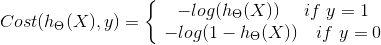若我们 令正样本的标签 y = 1 ，负样本的标签 y = -1，则有：其中（待续）七、代码示例使用ovr多分类的逻辑回归判断鸢尾属植物的类型from sklearn import datasets

X = iris.data
y = iris.target
print X.shape
print y.shape(150L, 4L)
(150L,)from sklearn.model_selection import train_test_split

#分隔训练集和测试集
X_train, X_test, y_train, y_test = train_test_split(X, y ,test_size = 1/3.,random_state = 8)from sklearn.preprocessing import PolynomialFeatures

featurizer = PolynomialFeatures(degree=2) # 特征多项式化
X_train = featurizer.fit_transform(X_train)
X_test = featurizer.transform(X_test)from sklearn.preprocessing import StandardScaler # 对数据归一化

scaler = StandardScaler()
X_std_train = scaler.fit_transform(X_train)
X_std_test = scaler.transform(X_test)from sklearn.linear_model import LogisticRegression
from sklearn.linear_model import SGDClassifier

# penalty:正则化 l2/l1
# C ：正则化强度
# multi_class:多分类时使用 ovr: one vs rest
lor = LogisticRegression(penalty='l1',C=100,multi_class='ovr')
lor.fit(X_std_train,y_train)
print lor.score(X_std_test,y_test)

sgdv = SGDClassifier(penalty='l1')
sgdv.fit(X_std_train,y_train)
print sgdv.score(X_std_test,y_test)0.94
0.92

LogisticRegression对参数的计算采用精确解析的方式，计算时间长但模型的性能高；SGDClassifier采用随机梯度下降/上升算法估计模型的参数，计算时间短但模型的性能较低。使用Tensorflow实现线性逻辑回归：from sklearn.datasets import make_classification
import matplotlib.pyplot as plt
import numpy as npX, y = make_classification(n_features=2, n_redundant=0, n_informative=2,
n_clusters_per_class=1,random_state=78,n_samples=200)
X = X.astype(np.float32)
y = y.astype(np.float32)plt.scatter(X[:,0],X[:,1],c=y)
plt.show()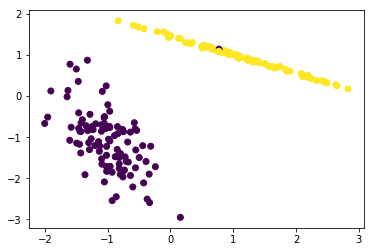from sklearn import preprocessing
scaler = preprocessing.StandardScaler().fit(X)
X = scaler.transform(X)
print X.shape(200, 2)b = tf.Variable(tf.zeros([1,1]))
W = tf.Variable(tf.zeros([2,1]))
X_DATA = tf.placeholder(tf.float32,[None,2])
Y = tf.placeholder(tf.float32,[None,1])H = 1 / (1 + tf.exp(-(tf.matmul(X_DATA, W) + b)))
loss = tf.reduce_mean(- Y*  tf.log(tf.clip_by_value(H,1e-11,1.0)) - (1 - Y) * tf.log(1 - tf.clip_by_value(H,1e-11,1.0)))
# 也可以使用tensorflow的版本：
#loss = tf.reduce_mean(tf.nn.sigmoid_cross_entropy_with_logits(labels=tf_Y,logits=pred))optimizer = tf.train.GradientDescentOptimizer(0.1)train = optimizer.minimize(loss)init_vals = tf.global_variables_initializer()
with tf.Session() as sess:
sess.run(init_vals)
for step in range(15501):
sess.run(train,feed_dict={X_DATA:X,Y:y.reshape(-1,1)})
if(step%5000==0):
print(step,sess.run(W).flatten(),sess.run(b).flatten())
w1 = sess.run(W).flatten()
b1 = sess.run(b).flatten()(0, array([ 0.04289575,  0.04343094], dtype=float32), array([-0.0005], dtype=float32))
(5000, array([ 3.44468737,  3.617342  ], dtype=float32), array([-1.10549724], dtype=float32))
(10000, array([ 3.46032   ,  4.07498837], dtype=float32), array([-1.60735476], dtype=float32))
(15000, array([ 3.45384622,  4.39454508], dtype=float32), array([-1.95797122], dtype=float32))print (w1,b1)(array([ 3.45412397,  4.42197132], dtype=float32), array([-1.9879719], dtype=float32))x1_min, x1_max = X[:,0].min(), X[:,0].max(),
x2_min, x2_max = X[:,1].min(), X[:,1].max(),
xx1, xx2 = np.meshgrid(np.linspace(x1_min, x1_max), np.linspace(x2_min, x2_max))
f = w1*xx1+w1*xx2+b1
plt.contour(xx1, xx2, f, , colors = 'r') # 绘制分隔超平面
plt.scatter(X[:,0],X[:,1],c=y)
plt.show()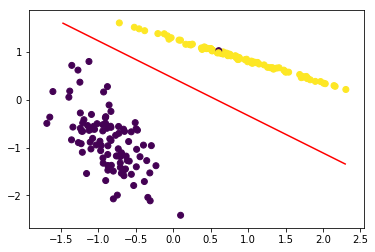展开全文• 在之前的文章中，介绍了神经网络的概念和算法思想，甚至给出了公式推导。但依然没有掌握神经网络的精髓，于是打算进一步学习就在网上观看了吴恩达大佬的《神经网络和深度学习》这门课程，觉得收获很大。...
       在之前的文章中，介绍了神经网络的概念和算法思想，甚至给出了公式推导。但依然没有掌握神经网络的精髓，于是打算进一步学习就在网上观看了吴恩达大佬的《神经网络和深度学习》这门课程，觉得收获很大。目前只学习了单个神经元的原理及应用，下面简单总结一下。1. 损失函数的定义       与之前介绍的单个神经元的工作原理不同之处在于损失函数的定义，这里把损失函数定义为：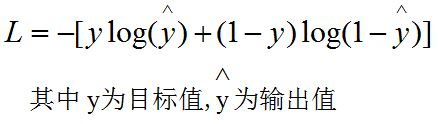推导思路：利用最大似然估计。先表达出p(y|x)，然后求其最大值。大致推导过程如下：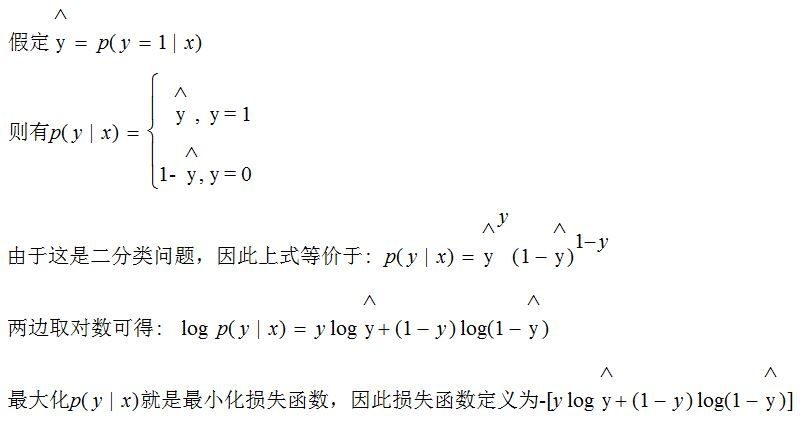课程中另一个重点是“消除for循环”，也就是说用向量来代替显示的for循环，这样做的好处是提高计算效率。实验测试100万条测试数据，向量化要比循环快上300倍。因此在以后的编码中，尽量把数据初始化成一个矩阵来处理。在这里值得注意的是，构造的矩阵应该每一列表示一个测试样例。2. 代码实现        下面给出基于神经网络的二分类代码（python）：import numpy as np
import h5py
import math

train_dataset = h5py.File('datasets/train_catvnoncat.h5', "r")
train_set_x_orig = np.array(train_dataset["train_set_x"][:]) # your train set features
train_set_y_orig = np.array(train_dataset["train_set_y"][:]) # your train set labels

test_dataset = h5py.File('datasets/test_catvnoncat.h5', "r")
test_set_x_orig = np.array(test_dataset["test_set_x"][:]) # your test set features
test_set_y_orig = np.array(test_dataset["test_set_y"][:]) # your test set labels

classes = np.array(test_dataset["list_classes"][:]) # the list of classes

train_set_y_orig = train_set_y_orig.reshape((1, train_set_y_orig.shape))
test_set_y_orig = test_set_y_orig.reshape((1, test_set_y_orig.shape))

return train_set_x_orig, train_set_y_orig, test_set_x_orig, test_set_y_orig, classes

def train_model(learning_rate=0.1):
train_data_x, train_data_y, test_data_x, test_data_y, classes = load_dataset()  # 获取数据集

train_data_x = train_data_x.reshape(209, 64*64*3).T # 把训练数据构造成二维矩阵，行数为X的维度，列值为训练样本的个数

row_count = 64*64*3 # 表示一个样例的维度
examples_count = 209 # 表示样例的数量

weight = np.zeros((64*64*3, 1)) # 初始化权值向量为0
b = 0 # 初始化阈值为0
dw = 0
db = 0
for i in range(1000):
z = np.dot(weight.T, train_data_x) + b # 计算z
y_hat = 1.0 / (1 + math.e**(-z)) # 计算预测值
result = np.sum(abs(y_hat - train_data_y )) # 以累加y_hat-y的值来表示预测效果，result越小越好
if result == 0:
break
print '%d:%d' %(i, result)

dz = y_hat - train_data_y # 计算dL/dz
dw = np.dot(train_data_x, dz.T) # 计算dL/dw
db = np.sum(dz)
dw = dw * 1.0 / examples_count
db = db * 1.0 / examples_count
weight = weight - learning_rate * dw
b = b - learning_rate * db

return weight, b

def predict_data(weight, b):
train_data_x, train_data_y, test_data_x, test_data_y, classes = load_dataset()  # 获取数据集

row_count = 64 * 64 * 3 # 一个样例的维度
example_count = 50 # 测试样例个数

test_data_x = test_data_x.reshape(example_count, row_count).T # 转换成对应格式的矩阵
z = np.dot(weight.T, test_data_x) + b
predict_y = 1.0 / (1 + math.e**(-z)) # 计算预测值
print 'correct rate:',np.sum(predict_y == test_data_y)* 1.0 / example_count
print predict_y

if __name__ == '__main__':
train_data_x, train_data_y, test_data_x, test_data_y, classes = load_dataset()  # 获取数据集
weight, b = train_model(learning_rate = 0.1) # 训练参数
predict_data(weight, b) # 根据训练的参数进行预测

       这里有一点需要注意，在构造矩阵的时候"test_data_x = test_data_x.reshape(example_count, row_count).T"并不等价于"test_data_x = test_data_x.reshape(row_count,example_count)"，如果直接用第二个语句，则会打乱原来每个样例的数据，构造的矩阵毫无实际意义。       在该测试样例中，训练时大概需要迭代600多次即可收敛，最后预测的准确率约为：72%。
展开全文神经网络 二分类
• 对于维度为m+1m+1m+1特征为xxx样本的二分类问题，有负类（Negative Class）记为000，正类（Positive Class）记为111，即对于类别yyy，有 y∈{0,1}.y∈{0,1}. y\in\{0,1\}. 我们期望找到一个hypothesis functionhθ...
问题描述

对于维度为m+1$m+1$$m+1$特征为x$x$$x$样本的二分类问题，有负类（Negative Class）记为0$0$$0$，正类（Positive Class）记为1$1$$1$，即对于类别y$y$$y$，有
y∈{0,1}.$y\in \left\{0,1\right\}.$ y\in\{0,1\}.
我们期望找到一个hθ(x)${h}_{\theta }\left(x\right)$$h_\theta(x)$，使得
0⩽hθ(x)⩽1.$0⩽{h}_{\theta }\left(x\right)⩽1.$ 0 \leqslant h_\theta(x)\leqslant 1 .
其中，θ$\theta$$\theta$为待优化的参数，使得在对未知类别的样本x0${x}_{0}$$x_0$分类时，
hθ(x0)${h}_{\theta }\left({x}_{0}\right)$$h_\theta(x_0)$为样本为正类的概率。即分类准则如下：
y0={0,1,if hθ(x0)<0.5;if hθ(x0)≥0.5.
y_0=\begin{cases}
0, & \text{if } h_\theta(x_0) <0.5;\\
1, & \text{if }  h_\theta(x_0) \ge 0.5.
\end{cases}

Logistic回归

在线性回归（Linear Regression）中，我们常找一组参数
θ=⎛⎝⎜⎜⎜θ0θ1...θm⎞⎠⎟⎟⎟$\theta =\left(\begin{array}{c}{\theta }_{0}\\ {\theta }_{1}\\ ...\\ {\theta }_{m}\end{array}\right)$\theta= \begin{pmatrix}
\theta_0 \\
\theta_1 \\
... \\
\theta_m
\end{pmatrix}
计算
f(x)=θTx.$f\left(x\right)={\theta }^{\mathrm{T}}x.$f(x)=\theta^\mathrm{T}x.
设置阈值T$T$$T$，通过f(x)$f\left(x\right)$$f(x)$与T$T$$T$的大小关系判断正负类。
而在Logistic回归中，我们引入Sigmoid函数
g(z)=11+e−z.$g\left(z\right)=\frac{1}{1+{e}^{-z}}.$g(z)=\frac{1}{1+e^ {-z}}.
其图像如下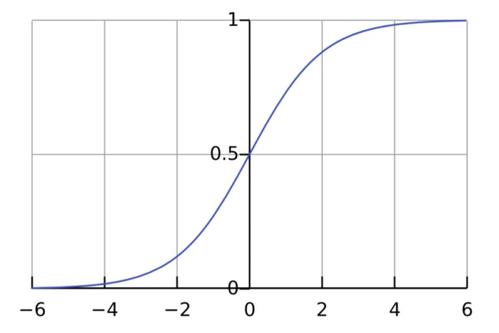Logistic回归取hypothesis function为
hθ(x)=g(θTx)=11+eθTx=p(y=1|x;θ)=p(y=0|x;θ).$\begin{array}{rl}{h}_{\theta }\left(x\right)& =g\left({\theta }^{\mathrm{T}}x\right)\\ & =\frac{1}{1+{e}^{{\theta }^{\mathrm{T}}x}}\\ & =p\left(y=1|x;\theta \right)\\ & =p\left(y=0|x;\theta \right).\end{array}$\begin{align*}
h_\theta(x) &= g(\theta^\mathrm{T}x)\\
&= \frac{1}{1+e^{\theta^\mathrm{T}x}}\\
&= p(y=1|x;\theta) \\
&= p(y=0|x;\theta).
\end{align*}
即hθ(x)${h}_{\theta }\left(x\right)$$h_\theta(x)$等价于正类的概率，由Sigmoid函数图像可知，当θTx≥0${\theta }^{\mathrm{T}}x\ge 0$$\theta^\mathrm{T}x\ge0$时，判定为正类，当θTx<0${\theta }^{\mathrm{T}}x<0$$\theta^\mathrm{T}x<0$时，判定为负类。

代价函数（cost function）

与线性回归问题类似，Logistic同样需要定义代价函数使用梯度下降法优化参数
由于Sigmoid函数的使用，若使用与线性回归相同的二次损失函数，优化问题将变为非凸问题，即可能存在很多局部最优解。Logistic回归采用以下损失函数
cost(hθ(x),y)={−log(hθ(x)),−log(1−hθ(x)),if y=1;if y=0.cost(h_\theta(x),y)= \begin{cases}
-\log(h_\theta(x)), &\text{if } y=1; \\
-\log(1-h_\theta(x)), &\text{if } y=0.
\end{cases}
为了方便计算，将分段损失函数改写为如下形式
cost(hθ(x),y)=−ylog(hθ(x))−(1−y)log(1−hθ(x)).$cost\left({h}_{\theta }\left(x\right),y\right)=-y\mathrm{log}\left({h}_{\theta }\left(x\right)\right)-\left(1-y\right)\mathrm{log}\left(1-{h}_{\theta }\left(x\right)\right).$
cost(h_\theta(x),y)=-y\log(h_\theta(x))-(1-y)\log(1-h_\theta(x)).

优化目标

对于样本数目为n$n$$n$的训练集，定义目标函数为
J(θ)=1n∑i=1ncost(hθ(x(i)),y(i))=−1n[∑i=1ny(i)log(hθ(x(i))+(1−y(i))log(1−hθ(x(i))]$\begin{array}{rl}J\left(\theta \right)& =\frac{1}{n}\sum _{i=1}^{n}cost\left({h}_{\theta }\left({x}^{\left(i\right)}\right),{y}^{\left(i\right)}\right)\\ & =-\frac{1}{n}\left[\sum _{i=1}^{n}{y}^{\left(i\right)}\mathrm{log}\left({h}_{\theta }\left({x}^{\left(i\right)}\right)+\left(1-{y}^{\left(i\right)}\right)\mathrm{log}\left(1-{h}_{\theta }\left({x}^{\left(i\right)}\right)\right]\end{array}$\begin{align*}
J(\theta) &=\frac1n \sum_{i=1}^n cost(h_\theta(x^{(i)}),y^{(i)})\\
&= -\frac1n \left[\sum_{i=1}^n y^{(i)}\log(h_\theta (x^{(i)})+(1-y^{(i)})\log(1- h_\theta (x^{(i)})\right]
\end{align*}
优化目标为：找到令J(θ)$J\left(\theta \right)$$J(\theta)$最小的θ$\theta$$\theta$.

算法描述

want$want$$want$ :          minθJ(θ)$mi{n}_{\theta }J\left(\theta \right)$min_\theta J(\theta)
Repeat$Repeat$$Repeat$
θj:=θj−α∂J(θ)θj,j=0,...,m.${\theta }_{j}:={\theta }_{j}-\alpha \frac{\mathrm{\partial }J\left(\theta \right)}{{\theta }_{j}},j=0,...,m.$\theta_j: = \theta_j - \alpha \frac{\partial J(\theta)}{\theta_j},j=0,...,m.

其中，α$\alpha$$\alpha$为梯度下降法的学习率.

优化算法列举

3）BFGS
4）L-BFGS

利用Octave实现Logistic回归

Octave是一种高层解释类编程语言，旨在解决线性和非线性的数值计算问题。Octave为GNU项目下的开源软件，早期版本为命令行交互方式，4.0.0版本发布基于QT编写的GUI交互界面。Octave语法与Matlab语法非常接近，可以很容易的将matlab程序移植到Octave。同时与C++,QT等接口较Matlab更加方便。
注：Octave与Matlab语法类似，下标从1开始。
例子：
θ=(θ1θ2)$\theta =\left(\begin{array}{c}{\theta }_{1}\\ {\theta }_{2}\end{array}\right)$\theta= \begin{pmatrix}
\theta_1 \\
\theta_2
\end{pmatrix}
J(θ)=(θ1−1)2+(θ2−1)2$J\left(\theta \right)=\left({\theta }_{1}-1{\right)}^{2}+\left({\theta }_{2}-1{\right)}^{2}$J(\theta)=(\theta_1-1)^2+(\theta_2-1)^2
∂J(θ)θ1=2(θ1−1)$\frac{\mathrm{\partial }J\left(\theta \right)}{{\theta }_{1}}=2\left({\theta }_{1}-1\right)$\frac{\partial J(\theta)}{\theta_1}=2(\theta_1-1)
∂J(θ)θ2=2(θ2−1)$\frac{\mathrm{\partial }J\left(\theta \right)}{{\theta }_{2}}=2\left({\theta }_{2}-1\right)$\frac{\partial J(\theta)}{\theta_2}=2(\theta_2-1)
代码：
定义函数，给出优化目标及对应的梯度，初始化梯度

    function [jVal, gradient] = costFunction(theta)
jVal = (theta(1)-1)^2 + (theta(2)-1)^2;
endfunction

设定option,设置梯度目标参数为打开，最大迭代次数为100，并初始化θ$\theta$$\theta$.

options = optimset('GradObj', 'on', 'MaxIter','100')
initialTheta = zeros(2,1)

调用fminunc这个函数，传入三个参数，其中第一个参数@costFunction这里的@符号代表指向之前我们定义的costFunction函数的指针。后面两个参数分别是我们定义的thetatheta初始值和配置信息options。它会自动的从众多高级优化算法中挑选一个来使用。

[optTheta, functionVal, exitFlag]=...
fminunc(@costFunction, initialTheta, options)

输出结果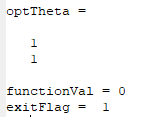即θ1=1${\theta }_{1}=1$$\theta_1=1$,θ2=1${\theta }_{2}=1$$\theta_2=1$，exitFlag=1$exitFlag=1$$exitFlag=1$表明已经收敛.

注：本文内容为网易云课堂吴恩达机器学习视频学习时的记录的笔记，仅做学习使用，笔者对OCTAVE首次接触，仅仅实现了课堂上的例子。如有错误，欢迎联系笔者。
展开全文机器学习 逻辑回归
• 解决思路 整体上采用迁移学习来训练神经网络，使用InceptionV3结构，框架采用keras. 具体思路： 读取图片数据，保存成.npy格式，方便后续加载 标签采用one-hot形式，由于标签隐藏在文件夹命名中，所以需要自行...
解决思路

整体上采用迁移学习来训练神经网络，使用InceptionV3结构，框架采用keras.

具体思路：

读取图片数据，保存成.npy格式，方便后续加载
标签采用one-hot形式，由于标签隐藏在文件夹命名中，所以需要自行添加标签，并保存到.npy文件中，方便后续加载
将数据分为训练集、验证集、测试集
使用keras建立InceptionV3基本模型，不包括顶层，使用预训练权重，在基本模型的基础上自定义几层神经网络，得到最后的模型，对模型进行训练
优化模型，调整超参数，提高准确率
在测试集上对模型进行评估，使用精确率、召回率
对单张图片进行预测，并输出每种类别的概率
知识点总结

如何加载实际数据，如何保存成npy文件，如何打乱数据，如何划分数据，如何进行交叉验证
如何使用keras进行迁移学习
keras中数据增强、回调函数的使用，回调函数涉及：学习速率调整、保存最好模型、tensorboard可视化
如何使用sklearn计算准确率，精确率，召回率，F1_score
如何对单张图片进行预测，并打印分类概率
如何指定特定GPU训练，如何指定使用GPU的内存情况

展开全文• ## 二分类问题

千次阅读 2018-11-23 14:30:06
1、分类的用途 2、分类的假设函数选取 h(x) 3、分类的决策边界（使得拟合过程更加具体，直观） 4、代价函数 cost function 的表示 J(teta） 5、迭代取最优的参数 一、分类的用途 相比于回归问题，分类问题得到的 是 ...
• 一、这个IMDB数据集包含了50000条电影评论，其中25000条用于训练，另外25000条用于测试。其label只包含0或1，其中0表示负面评价，1表示正面评价 from keras.datasets import imdb (train_data,train_labels),...keras 二分类
• UCI经典分类二分类数据集，机器学习算法测试。亲测可用。
• 数据集：泰坦尼克号的数据集 package ... import org.apache.spark.mllib.classification.{LogisticRegressionModel, LogisticRegressionWithSGD, SVMModel, SVMWithSGD} import org.apache.spark....
• 多分类问题下的评价指标分析（Precision, Recall, F1）二分类下的评价指标Accuracy几个常用的术语PrecisionRecallF1-score 二分类下的评价指标 Accuracy 分类算法有很多，不同分类算法有很多不同的变种。不同的分类...
• # -*- coding: utf-8 -*- import os import random import shutil #功能：创建文件夹 #传入参数：被创建文件夹的名字 def makedir(new_dir): if not os.path.exists(new_dir): os.makedirs(new_dir) ...
• ## 使用TensorFlow实现二分类

万次阅读 热门讨论 2018-09-21 08:20:48
使用TensorFlow构建一个神经网络来实现二分类，主要包括输入数据格式、隐藏层数的定义、损失函数的选择、优化函数的选择、输出层。下面通过numpy来随机生成一组数据，通过定义一种正负样本的区别，通过TensorFlow来...
• 多分类问题的基本思路是“拆解法”，将多分类任务拆为若干个二分类任务求解。经典的拆分策略有三种：一对多、一对其余和多对多。 二、拆分方法 1、 将个类别两两配对，形成个二分类任务。在测试阶段，新样本被...
• 二分类、多分类与多标签的基本概念 二分类：表示分类任务中有两个类别，比如我们想识别一幅图片是不是猫。也就是说，训练一个分类器，输入一幅图片，用特征向量x表示，输出是不是猫，用y=0或1表示。二类分类是假设...
• 对于二分类问题，可将样例根据其真实类别和分类器预测类别划分为： 真正例（True Positive，TP）：真实类别为正例，预测类别为正例。 假正例（False Positive，FP）：真实类别为负例，预测类别为正例。 假负例...
• 二分类问题评价指标评价指标准确率精确率召回率F1值ROCAUC 评价指标 二分类问题评价指标的相关整理，持续更新。 评价指标是针对同样的数据，输入不同的算法，或者输入相同的算法但参数不同而给出这个算法或者参数...机器学习 二分类问题评价指标
• 逻辑回归(二分类)是一种广义的线性回归(同样存在过拟合的可能)，逻辑回归的目标值通过sigmoid函数转换成0-1之间的概率值，以0.5作为阈值变成二分类问题。 （二分类问题的概率与自变量之间的关系图形往往是一个S型...Python 二分类 逻辑回归
• 本文通过对这些指标的原理做一个简单的介绍，然后用python分别实现二分类和多分类的ROC曲线。 1 基本概念 一个分类模型(分类器)是一个将某个实例映射到一个特定类的过程.分类器的结果可以是给出该实例所属的...ROC python 机器学习
• ## 2.二分类模型

千次阅读 2019-05-11 11:43:51
逻辑回归：一个用于二分类的算法 以猫的识别为例 图片（64X64像素）在计算机中的保存：三个64X64的矩阵,对应R,G,B三种像素的强度值。定义一个特征向量，线性存储所有像素值，总维度是64X64X3。 输入...吴恩达 深度学习 二分类
• 二分类问题的交叉熵损失函数;  在二分类问题中，损失函数为交叉熵损失函数。对于样本（x,y）来讲，x为样本 y为对应的标签。在二分类问题中，其取值的集合可能为{0，1}，我们假设某个样本的真实标签为yt，该样本的...
• 博主最近为了准备校招猛看有关gbdt的算法细节，有一个百思不得其解的疑惑，就是xgboost在处理二分类问题时，返回的预测值是0~1之间的概率值，莫非和logistic function有关？ 最终，我查阅了xgboost官网的介绍，得到...xgboost gbdt 二分类
...